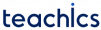# Multidimensional arrays in C

In c programming, multidimensional arrays can be simply defined as arrays of arrays. Multidimensional arrays store data in tabular form.

### Declaring Multidimensional Arrays in C

The declaration of an n-dimensional array takes the following form,

`dataType arrayName[size1][size2] ... [sizeN];`

For example,

`int A;`

Here A is a 2-dimensional array with 3 rows and 5 columns. The total number of elements that can be stored in a multidimensional array is the product of the size of all the dimensions. The size of this two-dimensional array will be 15.

Elements in this array can be referred to by A[i][j] where i is the row number and j is the column number.

A simple example of a 2-dimensional array having 3 rows and 5 columns is given below.

Here `A = 5`, `A = 3`, `A = 10`, `A = 8` and `A=15`.
Similarly, `A = 11`, `A = 19`, `A = 7`, `A = 1`, `A = 4` and so on.

### Initializing Multidimensional Arrays

A 2-dimensional array can be initialized in any of the following ways:

```int A = { {5, 3, 10, 8, 15}, {11, 19, 7, 1, 4}, {8, 9, 2, 3, 5} };

int A[] = { {5, 3, 10, 8, 15}, {11, 19, 7, 1, 4}, {8, 9, 2, 3, 5} };

int A = {5, 3, 10, 8, 15, 11, 19, 7, 1, 4, 8, 9, 2, 3, 5};```

It will create an array of the following form:

A 3-dimensional array can be initialized in a similar way. For example

```int A = {
{{1,7,8,2},{5,6,1,0}},
{{5,3,0,2},{4,6,1,8}},
{{8,6,2,1},{8,4,0,3}}};```

### Example: Sum of two matrices

```// finding the sum of two matrices of order 3*2

#include <stdio.h>
int main()
{
int a, b, sum;

// Getting elements of 1st matrix
printf("Enter elements of 1st matrix\n");
for (int i = 0; i < 3; ++i)
for (int j = 0; j < 2; ++j)
{
printf("Enter a%d%d: ", i + 1, j + 1);
scanf("%d", &a[i][j]);
}

// Getting elements of 2nd matrix
printf("Enter elements of 2nd matrix\n");
for (int i = 0; i < 3; ++i)
for (int j = 0; j < 2; ++j)
{
printf("Enter b%d%d: ", i + 1, j + 1);
scanf("%d", &b[i][j]);
}

// finding sum
for (int i = 0; i < 3; ++i)
for (int j = 0; j < 2; ++j)
{
sum[i][j] = a[i][j] + b[i][j];
}

// Displaying the resultant matrix
printf("\nSum Of Matrix\n");

for (int i = 0; i < 3; ++i)
{
for (int j = 0; j < 2; ++j)
{
printf("%d\t", sum[i][j]);
}
printf("\n");
}
return 0;
}```
##### Output
```Enter elements of 1st matrix
Enter a11: 1
Enter a12: 6
Enter a21: 2
Enter a22: 8
Enter a31: 0
Enter a32: 3
Enter elements of 2nd matrix
Enter b11: -3
Enter b12: 5
Enter b21: 9
Enter b22: 0
Enter b31: 2
Enter b32: 3

Sum Of Matrix
-2      11
11      8
2       6```
Subscribe
Notify of